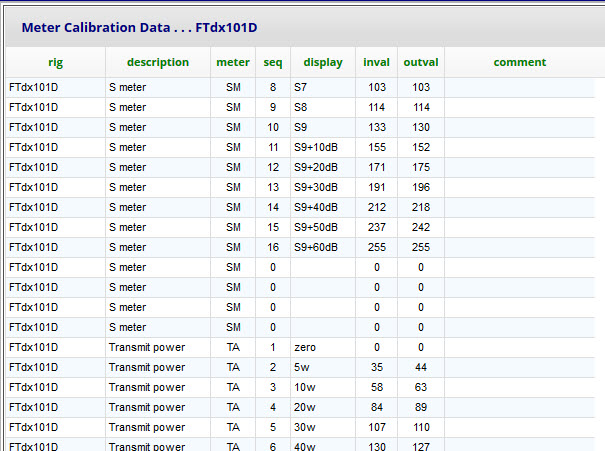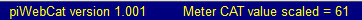9.2  S meter and Tx meter calibration

Below is a section of my metercal table for the FTdx101DThe table contains 120 records per radio   =  20 calibration points for each of six meters.

I strongly suggest that you do not need to modify the layout.
Simply enter the calibration data for you radio's selected meters.

If the meter falls back to zero at full scale, then add a final extra record:
eg:  seq = 17   inval = 255    outval = 255

The S meter has meter = SM.  The Tx meters have meter = TA, TB, TC, TD and TE.

(Note that meter function and button captions are defined in meter or meterciv table.)

In the meter and meterciv tables, each meter has mult and divide for optional linear scaling

This scaling can be used for pre-calibration (eg 0-255 to 0-100)

This scaling happens before the calibration table is applied.

The meter CAT data on the page footer is AFTER the scaling.

Each meter can have up to 20 calibration points.

The seq field defines the order (needed for interpolation),  Unused points have seq = 0.

Calibration procedure:   uses the CAT value on the footer barThe meter's usecal field in the meter or meterciv table must be set to N  ( = no!).

eg: S meter - use RF gain control.   Tx power -  CW to dummy load.

Example - S meter:

- first entry inval = 0 outval = 0

- then set S1 level on the radio and record CAT data for inval.

- then set S1 level on piWebCAT and record CAT data for outval

- repeat for S2 ... S9 and +10dB ... + 50dB etc.

- Enter the collected values into the metercal table as above.

Finally set usecal in meter or meterciv table to Y to test and use the new calibration.